• 连续非周期信号频谱分析及Matlab 实现
千次阅读
2021-04-30 00:38:36连续非周期信号频谱分析及Matlab 实现

连续非周期信号频谱分析及 MATLAB 实现 谢海霞 1，孙志雄 1 (琼州学院海南 三亚 572022) 摘要：为了便于计算机辅助计算复杂的连续信号频谱，常常采用DFT方法。DFT不仅能反映信号的频域特征更便于用计算机处理。这里先对连续非周期信号做离散化处理，然后截短得到有限长序列，最后做DFT变换。针对常用信号DFT谱分析的原理及谱分析中的相关问题进行了较为深入的探讨，并结合实例用Matlab 仿真软件进行了分析和验证。 关键词：DFT；频谱分析；MATLAB 中图分类号：TN911.72 文献标识码：A Continuous-Time Aperiodic Signal Spectrum Analysis Based on MATLAB XIE Haixia1 SUN Zhixiong1 (Qiongzhou University sanya Hainan 572022 China) Abstract: In order to calculate the spectrum of complex continuous signal with the computer the DFT is normally used. DFT can not only reflect the characteristic of the signal in the frequency domain is moreconvenient for processing by computer. Here do discretization continuous-time aperiodic signal first then cut shortby finite length sequences，finally DFT trans.This paper has made a deep discuss in the principle and somerelated questions of DFT in signal spectrum analysis. Matlab simulation software is used to analyze and test thedesign. Key words: Discrete Fourier trans(DFT) spectrum analysisMATLAB 算法，便于用计算机处理。4-6本文介绍具体的连0 引 言 续非周期信号结合 MATLAB 软件来分析其频谱。 频谱分析在数字信号处理中用途广泛：如滤波、检测等方面，这些都需要 DFT(Discrete Fourier 1 连续非周期信号谱 DFT 分析 Trans)运算。1-3信号的 Fourier 变换建立了 在已知连续信号数学解析式的情况下，非周期信号的时域与频域之间的一一对应关系，信号的 信号的频谱可以根据 Fourier 变换的定 义进行解析Fourier 变换可以借助 DFT 来分析。有限长序列的 计算。实际应用中的多数信号不存在数学解析式，DFT 可以由数字方法直接计算，有 DFT 存在快速 信号的频谱无法利用傅里叶分析公式方法直接计 算，一般需采用数值方法进行近似计算分析频谱，基金项目：海南省自然科学基金项目611133。作者简介：谢海霞(1978-)，女，讲师，工学硕士，主要研 在进行数字计算时，需对计算的连续变量进行离散究 EDA 技术在信号处理中的应用。 化。由于连续非周期信号 xt的频谱函数 Xjw是连 续信号一般都不是带限信号，连续信号在抽样前通续函数，因此，需要 对其进行离散化处理 xn以近 常都经过一个模拟低通滤波器(称为抗混叠滤波似分析相应的频谱。有限长序列的 DFT 可以由数 器)进行低通滤波，以减少混叠误差，以提高频谱字方法直接计算，且 DFT 存在快速算法。通过建 分析精度。 2.2 泄漏现象立序列 xn有离散 Fourier 变换 Xm与连续非周期 对连续非周期信号的采样序列 xn进行 DFT信号 xt 的 Fourier 变换 Xjw之间的关系，可以利 运算 时， 时间长度总是取有限值，在将信号截短即用 DFT 对连续非周期信号频谱进行近似分析，此 时域加窗处理的过程中，出现了分散的扩展谱线的近似分析过程中一般将会出现三种现象：混叠现 现象，称为频谱泄漏。对离散序列的加窗实际上是象、泄漏现象和栅栏现象。7-8这些现象与应用中 将离散序列与窗函数相乘，加窗后信号的频谱是加信号和 DFT 的参数选择有关。下面分别讨论近似 窗前信号的频谱与窗函数频谱的卷积，造成截短后过程中可能出现的问题及其解决方法。 信号的频谱与截短前信号的频谱不同，所得的频谱2 DFT 分析过程中出现的若干问 在原来没有频谱的区间出现了频谱。原来比较尖锐题 的谱峰变得比较平缓，当两个不同频率的谱峰靠得2.1 混叠现象 比较近时，可能显现不出两个明显的峰值。特 别是 由 DFT 计算出的频谱是信号 xt的频谱 Xjw 强信号谱的旁瓣可能淹没弱信号的主谱或误认为周期化的抽样值，如果连续信号不是带限信号，或 是另一假信号的主谱线。矩形窗的旁瓣幅度大，谱者抽样频率不满足抽样定理，在 连续信号离散化 间干扰严重。频谱泄漏使频谱变模糊，分辨率 变(事时，就会出 现信号频谱的混叠。解决连续信号离散 实上通常规定 DFT 的频率分辨率 为 fs/N， 为采样 fs化过程中的频谱混叠主要有两种方法：对于带限连 频率， N 是指信号 xn的有效长度)差，泄漏程度续信号，只要提高抽 样频率使之满足时域抽样定 与窗函数幅度谱主瓣宽度有关。窗型一定，窗口越理：对于非带限连续信号，可根据实际信号对其进 长，主瓣越窄，频谱泄漏越小，窗口 长度一定，矩行低通滤波，使之成为带限信号。工程实际中的连 形窗主瓣最窄，频谱泄漏最小，但其旁瓣的幅度最大。因此为了尽量减少泄漏现象，应选用旁瓣幅度 在利用 DFT 分析连续时间信号的频谱时，涉小、主瓣窄，即：“ 泄漏” 小的窗函数。相对而言，布 及频谱混叠、频率泄漏及栅栏现象。 频率混叠与连莱克曼窗的旁瓣幅度比矩形窗小，谱间干扰小，但 续信号的时域抽样间隔有关，频率泄漏与信号的时其主瓣过渡带宽，分辨率差。采 样频率或采样周期 域加窗截短的长度及窗型有关，栅栏现象与 DFT是在满足混叠误差前提下选取的，当采样频率或采 的点数有关。在大多数情况下，一般已知待分析连样周期确定后，适当增加窗口 长度有利于减小泄漏 续信号的最高频率，以及希望的 DFT 分析的频率误差。 分辨率。下面根据信号的 Fourier 变换的理论， 讨2.3 栅栏现象 论利用 DFT 进行谱分析的参数(抽样频率、持续 DFT 得到的频谱 Xm只能是连续非周期信号 时间、样点数等)选择的原则。 频谱 Xjw上的有限离散频点采样，由于 Xm是离 (a)首先确定信号抽 样频率 f s ， f s 应满足散序列，因而无法反映抽样点之间的细节，就如同 f m 为待分析 时域抽样定理，即 f s ≥ 2 f m ，其中隔着百叶窗观察窗外的景色，这种现象称为栅栏现 的连续信号的

更多相关内容
• 为了形象地呈现周期连续信号分解为若干谐波成分或由不同的谐波合成周期信号这一过程, 采用 M A TLAB 数值计算和 GU I 程序设计的方法和技巧, 编写了其图形用户界面程序。通过实例演示, 特别是能对 任意周期信号的...
• 学 海 无 涯 非周期信号的傅里叶变换MATLAB 仿真实验 一实验目的 1熟悉连续非周期信号频谱特点及其分析方法 2 掌握用MATLAB 实现傅里叶变换 二非周期信号的傅里叶变换原理及性质 设周期信号 f (t) 展开成复指数形式...
• 2020年TI杯全国大学生电子设计竞赛模拟电子系统专题邀请赛，赛题分享，A题单次周期信号再现装置。该题目是一道经典的模数结合的电子系统设计案例，考察学生信号调理、采集、处理和输出的能力。题目中既包含了平时...
• 在定义信号周期性的基础上，针对多个周期信号、多个周期信号和其他信号单通道混合的情况，提出了基于特征值分析的周期信号盲分离方法，并对算法的可行性、分离误差及分离效果进行了理论分析．仿真结果表明：该方法能...
• 基于Lab view的周期信号分解与合成实验研究利用 LabV IEW 软件可以很方便的将周期信号进行分解, 直观而形象的对周期信号的构成进行研究, 利用傅里叶级数中的有限次正弦谐波近似的合成周期信号。可以看到谐波次数越多...Labview
• 利用MATLAB GUI仿真电路周期信号的分解与合成.pdf
• 周期信号的傅里叶变换MATLAB仿真实验 实验目的 熟悉连续非周期信号频谱特点及其分析方法 掌握用MATLAB实现傅里叶变换 非周期信号的傅里叶变换原理及性质 设周期信号展开成复指数形式的傅里叶级数为 两边同乘 得 上...
• 周期单位脉冲序列的频谱 等间隔的周期单位脉冲序列也称为梳状函数，如图2.34(a)所示，表示为 (2-75) 式中， 为周期； 为整数， ； 为周期函数，根据式(2-74)有 (2-76) 式中， ，而系数由式 (2-21)确定，即 在区间( ,...
• 1.连续时间周期信号的傅里叶级数分析任何一个周期为T的正弦周期信号，只要满足狄利克里条件，就可以展开成傅里叶级数，(至于为什么能展开傅里叶级数和什么是狄利克利条件，这里先不说，我们知道有这样的结论就好)。...

1.连续时间周期信号的傅里叶级数分析

任何一个周期为T的正弦周期信号，只要满足狄利克里条件，就可以展开成傅里叶级数，(至于为什么能展开傅里叶级数和什么是狄利克利条件，这里先不说，我们知道有这样的结论就好)。三角形式的傅里叶级数为：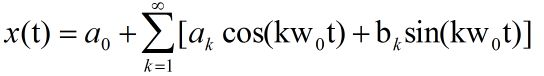或写成合并的形式：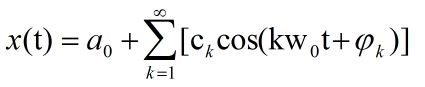其中(这里用括号代表下标)w(0)=2*pi/T,   a(0),a(k),b(k)分别代表直流分量，余弦分量幅度，正弦分量幅度，C(k),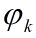为合并后的各正弦谐波分量的幅度和初相位，这两个都是kw(0)的函数，画出它们与kw(0)之间的关系的图像称为信号的频谱图，C(k)—kw(0)图像为幅度谱，—kw(0)为相位谱。

傅里叶级数就是说一个周期信号能够由无限个不同频率的正弦信号组成，这些正弦信号称为谐波分量，而频率随着k的增大而增大，k=1为一次谐波，k=2为二次谐波，可知谐波的次数越大，频率就越大(越往后的谐波分量在这个信号中占的份量就越小，即影响不大所以k可以取到有限)。也可以反过来理解：用无限个正弦谐波分量可以合并成一个任意的非正弦周期信号。(这就可以理解为什么会有用频带滤波器来消除噪音，所谓的噪音可看成是一个频率较小的谐波分量，加到信号上面就使信号变了样，所以这时候要去掉和噪音频率相近(因为不知道噪音频率是多少)的谐波分量就是频带滤波，同样的低通高通也一样，通过对相应的频率进行处理，只不过这时候就要换到频域上面才能进行滤波，上面说到的两个频谱图就是换到频域上的例子)。

由欧拉公式，可以把三角形式的傅里叶级数换成指数形式的傅里叶级数为：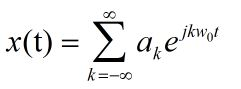这样周期信号也可以由无限个不同频率的互为谐波关系的周期复指数信号组成。

其中a(k)为指数形式的傅里叶级数的系数。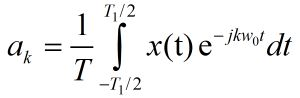其实系数a(k)与三角形式中的a(k),b(k)有关系，a(k)=1/2*(a(k)-jb(k)),所以a(k)为一个复数，绝对值为该谐波分量的幅度，相位角可由实数a和虚数b得到。(为什么会有正负呢？这完全是数学运算的结果，只有把负频率项与相应的正频率项成对地合并起来，才是实际的频谱函数。)

2.用MATLAB画出一个周期信号的频谱图

T=2;dt=0.0001;t=-2:dt:2;

>> x1=sin(t);

>> w0=2*pi/T;

>> N=10;

>> L=2*N+1;

>> for k=-N:N; %表示谐波分量

ak(N+1+k)=(1/T)*x1*exp(-j*k*w0*t')*dt;

end

>> phi=angle(ak);

>> subplot(211);

>> f=(-N:N)*w0

>> plot(f,abs(ak))

>> subplot(212);

>> plot(f,phi)

3.非周期信号的傅里叶变换分析

这里就不说明非周期信号的傅里叶变换怎么得来，怎么由离散频率变为连续频率了。直接来两条公式再对公式进行说明。

傅里叶变换和其逆变换：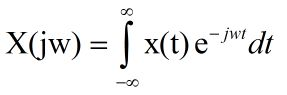，，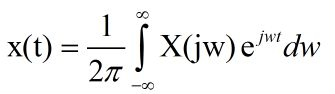任意非周期信号，如果满足狄利克里条件，那么，这个信号可以看成是由无穷多个不同频率(这些频率非常的接近，可看作连续)的周期复指数信号的线性组合构成的。每个频率对应的周期复指数信号称为频率分量，其相对幅度为对应频率的|X(jw)|的值，其相位为对应频率的相位X(jw)的相位。

MATLAB实现，由于计算机只能处理有限大小的数，所以只能取一定的值。

>> T=0.01;dw=0.1;

>> t=-10:T:10;

w=-10:dw:10;

for jw=w

X(w+11)=x1*exp(-j*t'*w)*T %计算傅里叶变换

Xf=abs(X); %计算幅度谱

phai = angle(X) %计算相位谱

写完这篇博客后，我发现了一篇知乎上的好文章http://zhuanlan.zhihu.com/wille/19759362

展开全文• 通过傅里叶级数展开，将周期信号分解为一系列“加权”的正弦信号的叠加，对傅里叶级数展开各个频率分量的系数CnC_nCn​，通过将周期信号的周期趋近无穷大，进而得到了非周期信号的傅里叶变换F(jω)F(j\omega)F(jω)...

在之前的分析中，通过傅里叶级数可以将周期信号展开为一系列“加权”正弦信号的叠加，而对傅里叶级数展开各个频率分量的系数 C n C_n ，通过将周期信号的周期趋近无穷大，进而得到了非周期信号的傅里叶变换 F ( j ω ) F(j\omega)

那么傅里叶变换是否可以用于周期信号呢？这样便可以将信号的分析方式统一起来，下面将对这个问题进行分析。

## 1. 如何得到周期信号的傅里叶变换？

首先，在求解非周期信号的傅里叶变换时，通过对周期信号的傅里叶级数的展开系数 C n C_n 进行分析和求解，进而得到非周期信号的傅里叶变换 F ( j ω ) F(j\omega) 。而周期信号的傅里叶变换则是通过对傅里叶级数展开式 f ( t ) = ∑ n = − ∞ + ∞ C n ⋅ e j n Ω t f(t)=\sum_{n=-\infty}^{+\infty}C_n\cdot e^{jn\Omega t} 两边同时求傅里叶变换得到。如何理解这样的求解方式呢？

在分析和求解周期信号的傅里叶变换之前，首先应了解下述两个问题：

1. 周期信号和非周期信号的相对性：傅里叶变换的由来是通过将周期信号的周期趋于无穷大而得到的，也就是说，周期和非周期信号可以理解为一个有限周期的周期信号和一个无限周期的周期信号。因此，非周期信号也可以看做是一种特殊的周期信号，反过来说，非周期信号也可以说是一种特殊的周期信号。因此傅里叶变换实际上也可以应用于对周期信号。这保障了傅里叶变换对周期信号进行变换的合理性
2. 回顾Direchlet的第一个条件：信号要在单个周期内或一段有限的时间范围内绝对可积，即 ∫ t 1 t 2 ∣ f ( t ) ∣ d t < ∞ \int_{t_1}^{t_2}|{f(t)|}dt<\infty 。傅里叶级数的求和范围是单个周期T或有限的时间区间 [ t 1 , t 2 ] [t_1, t_2] ，并且通过傅里叶级数将信号展开为谐波分量进行研究，而傅里叶变换是的积分范围是从 [ − ∞ , + ∞ ] [-\infty, +\infty] ，因此无法对周期信号进行傅里叶变换，不具备可行性。

为了解决上述的第二个问题，这里引入两个措施：

1. 傅里叶变换具备一个重要性质：线性特性

• 线性特性：信号的和的傅里叶变换等于傅里叶变换的和：即： F { A ⋅ f 1 ( t ) + B ⋅ f 2 ( t ) } = A ⋅ F [ f 1 ( t ) ] + B ⋅ F [ f 2 ( t ) ] F\{A\cdot f_1(t)+B\cdot f_2(t)\}=A\cdot F[f_1(t)]+B\cdot F[f_2(t)]
2. 复指数信号的傅里叶变换为： F [ e j ω c t ] → 2 π ⋅ δ ( ω − ω c ) F[e^{j\omega_c t} ] \rightarrow2\pi \cdot\delta(\omega-\omega_c) ，冲激函数可以实现将功率信号实现傅里叶变换。

一个满足Direchlet条件的周期信号一定可以展开为傅里叶级数，即：
f ( t ) = ∑ n = − ∞ + ∞ C n ⋅ e j n Ω t f(t)=\sum_{n=-\infty}^{+\infty}C_n\cdot e^{jn\Omega t}
其中系数 C n = 1 T ∫ − ∞ + ∞ f ( t ) ⋅ e − j n Ω t d t C_n = \frac{1}{T} \int_{-\infty}^{+\infty} f(t)\cdot e^{-jn\Omega t}dt Ω = 2 π T \Omega =\frac{2\pi}{T}

将周期信号看为相对的非周期信号，通过傅里叶变换的线性特性，可以将每一个 e j n Ω t e^{jn\Omega t} 求出傅里叶变换并乘以 C n C_n ，再叠加，便可得到原周期信号的傅里叶变换，如下：
F [ f ( t ) ] = ∑ n = − ∞ + ∞ C n ⋅ 2 π ⋅ δ ( ω − n Ω ) F[f(t)]=\sum_{n=-\infty}^{+\infty}C_n\cdot 2\pi \cdot \delta(\omega-n\Omega)
上式即周期函数的傅里叶变换。

通过上式可知：

1. 周期信号的傅里叶变换是一系列间隔均匀的冲激序列。
2. 幅度频谱仅出现在频域 ω = n Ω \omega =n\Omega 的点上.
3. 幅度的大小和信号对应傅里叶级数展开的系数 C n C_n 成正比，即 2 π ⋅ C n 2\pi \cdot C_n

所以，如果可以得到一个周期函数的额傅里叶级数展开式，那么这个周期函数的傅里叶变换，就是将原傅里叶级数展开频谱图上各个分量幅值乘以 2 π 2\pi

#### 问题：傅里叶级数和傅里叶变换对周期信号的分析有什么区别？

首先列出傅里叶级数和傅里叶变换的形式：

周期信号的傅里叶级数周期信号的傅里叶变换
f ( t ) = ∑ n = − ∞ + ∞ [ C n ⋅ e j ( n Ω t ) ] f(t)=\sum_{n=-\infty}^{+\infty}[C_n\cdot e^{j(n\Omega t)}] F [ f ( t ) ] = ∑ n = − ∞ + ∞ C n ⋅ 2 π ⋅ δ ( ω − n Ω ) F[f(t)]=\sum_{n=-\infty}^{+\infty}C_n\cdot 2\pi \cdot \delta(\omega-n\Omega)
C n = 1 T ∫ t 1 t 2 f ( t ) e − j n Ω t d t C_n = \frac{1}{T}\int_{t_1}^{t_2}f(t)e^{-jn\Omega t}dt C n = 1 T ∫ t 1 t 2 f ( t ) e − j n Ω t d t C_n = \frac{1}{T}\int_{t_1}^{t_2}f(t)e^{-jn\Omega t}dt
幅度频谱中各个分量的幅值为 C n C_n 幅度频谱中各个分量的幅值为 2 π ⋅ C n 2\pi \cdot C_n

观察上式，我认为主要区别如下：

1. 变换前后信号的域不同

• 周期信号的傅里叶级数是对周期性信号在时域的正交分解，所以傅里叶级数是一种时域到时域的分解，因此傅里叶级数本身并没有发生域的转化。
• 周期信号的傅里叶变换是对周期性信号表达方式的一种转化，将时域中信号的信息，反映在频域中。所以，周期性信号的傅里叶变换是一种时域到频域的转化
2. 分析的重点不同：

• 周期信号的傅里叶级数展开本质上是将一个信号通过正交函数集进行表示，表示的方式是利用线系统的叠加性和齐次性，对组成信号的子信号进行加权和叠加。而其系数 C n C_n 关于 n Ω n\Omega 的关系才是频域。因此傅里叶级数展开和傅里叶级数展开的频谱是两个概念。这里分析的重点是”展开“，而频谱只是对这种展开的一种表现形式，因为它可以很好体现展开式中的分量幅值、相位等信息。
• 周期信号的傅里叶变换本质上是构成信号的分量的幅值关于频率的函数，这对应于傅里叶级数中 C n C_n 关于 n Ω n\Omega 的关系。因此，周期信号的傅里叶变换，分析的重点是”变换“，即通过频域来体现一个信号的特点。
3. 频谱上的幅值不同：

• 周期信号的傅里叶级数，信号的各个频率分量出现在 n Ω n\Omega 的点上，其各个频率分量的幅值为傅里叶级数展开的系数 C n C_n
• 周期信号的傅里叶变换，信号的各个频率分量出现在 n Ω n\Omega 的点上，但其各个频率分量的幅值为傅里叶级数展开的系数 C n C_n 2 π 2\pi 倍，即 2 π ⋅ C n 2\pi \cdot C_n

#### 问题：周期信号的傅里叶变换、非周期信号的傅里叶变换，傅里叶级数有什么联系和区别？

1. 两者都是信号的各个频率分量幅值在频率上的体现。

2. 非周期信号的傅里叶变换是通过对周期信号的傅里叶级数展开的系数 C n C_n 进行分析和变化得到的。而周期信号的傅里叶变换，由于无法对 T T 取极限通过 C n C_n 求得，所以利用了傅里叶变换的线性性质，对傅里叶级数两边同时求傅里叶变换而得到的。

3. 周期信号的傅里叶级数的频谱是离散频谱，在除 n Ω n\Omega 之外的部分没有意义，而周期信号的傅里叶变换是连续频谱，在 n Ω n\Omega 上的频率有幅值，而在除 n Ω n\Omega 之外的部分为0，有意义。

#### 问题：如何理解”对 f ( t ) f(t) 进行傅里叶级数展开，或对 f ( t ) f(t) 进行傅里叶变换“这句话？

1. f ( t ) f(t) 进行傅里叶级数展开，是将 f ( t ) f(t) 用一系列正交函数（正弦函数）进行表示。
2. f ( t ) f(t) 进行傅里叶变换，是体现构成 f ( t ) f(t) 的各个频率分量的幅值在关于频率的关系，可以理解为特定条件和极限下的傅里叶级数展开系数的”变体“。

## 2. 周期延拓的信号的傅里叶变换

其实，周期延拓的信号的傅里叶变换，和周期信号的傅里叶变换是一样的，如果原非周期函数为 f ( t ) f(t) ，经过周期为 T T 的周期延拓，变为 f T ( t ) f_T(t) ，如果没有发生重叠的话， f T ( t ) f_T(t) 傅里叶变换为：
f T ( t ) = ∑ n − ∞ + ∞ C n ⋅ e j n Ω t → 2 π ∑ n − ∞ + ∞ [ C n ⋅ δ ( ω − n Ω ) ] \begin{aligned} &f_T(t)=\sum_{n-\infty}^{+\infty}C_n \cdot e^{jn\Omega t} \\&\rightarrow 2\pi \sum_{n-\infty}^{+\infty}[C_n\cdot \delta(\omega-n\Omega )] \end{aligned}

#### 问题：周期延拓后的 f T ( t ) f_T(t) 的傅里叶变换和原信号 f ( t ) f(t) 的傅里叶变换有什么关系？

通过对系数 C n C_n 进行计算，如下：
C n = 1 T ∫ − T 2 + T 2 f T ( t ) ⋅ e − j n Ω t d t = 1 T ∫ − ∞ + ∞ f ( t ) ⋅ e − j n Ω t d t = F ( j n Ω ) T \begin{aligned} C_n &= \frac{1}{T}\int_{-\frac{T}{2}}^{+\frac{T}{2}}f_T(t)\cdot e^{-jn\Omega t}dt \\&= \frac{1}{T}\int_{-\infty}^{+\infty}f(t)\cdot e^{-jn\Omega t}dt \\&=\frac{F(jn\Omega)}{T} \end{aligned}
所以：
f T ( t ) = ∑ n − ∞ + ∞ C n ⋅ e j n Ω t → 2 π ∑ n − ∞ + ∞ [ C n ⋅ δ ( ω − n Ω ) ] → 2 π T ∑ n − ∞ + ∞ [ F ( j n Ω ) ⋅ δ ( ω − n Ω ) ] → Ω ∑ n − ∞ + ∞ [ F ( j n Ω ) ⋅ δ ( ω − n Ω ) ] \begin{aligned} &f_T(t)=\sum_{n-\infty}^{+\infty}C_n \cdot e^{jn\Omega t} \\&\rightarrow 2\pi \sum_{n-\infty}^{+\infty}[C_n\cdot \delta(\omega-n\Omega )] \\&\rightarrow \frac{2\pi}{T} \sum_{n-\infty}^{+\infty}[F(jn\Omega)\cdot \delta(\omega-n\Omega )] \\&\rightarrow \Omega \sum_{n-\infty}^{+\infty}[F(jn\Omega)\cdot \delta(\omega-n\Omega )] \end{aligned}
也就是说，周期化的信号的傅里叶变换，是原信号的频谱的幅值乘以 Ω \Omega ，即 F ( j n Ω ) ⋅ Ω F(jn\Omega)\cdot \Omega ，再以 n Ω n\Omega 等间距对原信号频谱进行取样，如下图所示：## 3. 傅里叶级数，周期信号的傅里叶变换，非周期信号的傅里叶变换的求法

通过上述分析可知：

1. 非周期信号的傅里叶变换 – 直接计算或者查找傅里叶变换表得到 F ( j ω ) F(j\omega)
2. 周期信号的傅里叶变换 – 看为非周期信号 f ( t ) f(t) 的延拓 f T ( t ) f_T(t) ，通过 F [ f T ( t ) ] → Ω ∑ n − ∞ + ∞ [ F ( j n Ω ) ⋅ δ ( ω − n Ω ) ] F[f_T(t)]\rightarrow \Omega \sum_{n-\infty}^{+\infty}[F(jn\Omega)\cdot \delta(\omega-n\Omega )] 求得，其中 F ( j n Ω ) F(jn\Omega) 为非周期信号 f ( t ) f(t) 的傅里叶变换，并且在 n Ω n\Omega 取值。
3. 利用 C n = F ( j n Ω ) T C_n =\frac{F(jn\Omega)}{T} 求得周期信号的傅里叶级数。

所以，只需要知道非周期信号的傅里叶变换，便可以求得周期信号的傅里叶变换和傅里叶级数展开。

傅里叶变换和傅里叶级数的主要内容至此已经完成，下一部分将简略说明傅里叶变换的性质。感谢阅读，如有不当之处，欢迎批评指正！

展开全文• 该电路能够实现对准周期信号的整周期同步采样，具有倍频精度高、跟踪速度快、能对准周期信号进行预测和补偿等特点；同时介绍了一种周期预测的方法和原理以及基于PC总线实现准周期信号的同步数据采集系统。 关键词：...
• 在研究周期性振动信号处理方法的基础上,针对连续非周期信号难以有效识别的问题,提出了一种基于小波变换和自功率谱相结合的故障诊断方法,采用小波变换对源信号进行去噪处理,并对处理后的信号进行自功率谱分析,解决了...
• 题目片段：已知周期半波余弦信号和周期全波余弦信号的波形如图所示，用MATLAB编程求出它们的傅里叶系数，绘出其直流、一次、二次、三次、四次、及五次谐波叠加后的波形图，并将其与周期信号的时域波形进行比较，观察...
• 在这一篇 BlogBlogBlog 中，博主打算记录一下周期信号傅里叶级数的一些重要性质。在阅读本文之前，请明确一件事情：对于周期信号我们讲的是傅里叶级数展开。对于非周期信号我们讲傅里叶变换。 文章目录一、什么样的...

在这一篇 B l o g Blog 中，博主打算记录一下周期信号傅里叶级数的一些重要性质。在阅读本文之前，请明确一件事情：对于周期信号我们讲的是傅里叶级数展开。对于非周期信号我们讲傅里叶变换。

# 一、什么样的周期信号才能够做傅里叶展开？

当时傅里叶的论文中有这样一句话：“所有的连续时间信号都能够表示成成谐波关系的复指数信号的加权和”。在当时引来了像拉普拉斯等人的强烈反对。傅里叶认为：对于周期方波信号，只要我取的正弦信号足够多，那么我就一定能够完美地拟合出来。如下图所示：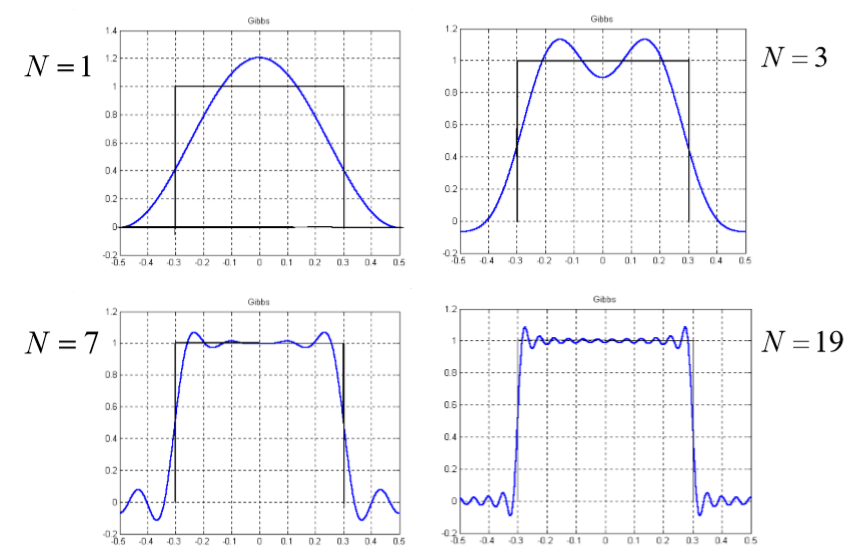但实际上并不是这样，无论我们的正弦信号取了多少，在原方波的间断点处不可避免地会出现震荡和超量。超量的幅度并不会随着我们所选取的正弦波的数量增多而减少，选取的正弦波的数量增多只会使得超量的震荡频率更大，并且朝着间断点处压缩。这就是所谓的 “吉布斯现象”。

那么，到底什么样的连续时间信号才能够展开成傅里叶级数呢？我们下面给出三个条件（其中条件三是狄里克雷第一定理）。连续时间的周期信号只要满足三者之一，就可以展开成傅里叶级数：
【条件一】：信号全部连续
【条件二】：信号在一个周期内能量有限： 1 T 0 ∫ T 0 ∣ x ( t ) ∣ 2 d t < ∞ \frac{1}{T_0}\int_{T_0}|x(t)|^2dt <∞
【条件三】：信号在一个周期内绝对可积： 1 T 0 ∫ T 0 ∣ x ( t ) ∣ < ∞ \frac{1}{T_0}\int_{T_0}|x(t)|<∞

# 二、周期信号傅里叶级数的重要性质

## 2.1 线性

首先我们给出定义：

连续时间信号傅里叶级数的线性性：若信号 x 1 ( t ) x_1(t) 的傅里叶级数是 a k a_k ，信号 x 2 ( t ) x_2(t) 的傅里叶级数是 b k b_k ，那么，信号 A x 1 ( t ) + B x 2 ( t ) Ax_1(t) + Bx_2(t) 的傅里叶级数就是 A a k + B b k Aa_k + Bb_k

首先，我们先知道求傅里叶系数的过程就是一个积分的过程，如果我们用 ∫ \int 表示计算傅里叶系数（当然这样的写法不够准确，但是为了说明问题暂且先这样用）。

所以，我们如果对信号 A x 1 ( t ) + B x 2 ( t ) Ax_1(t) + Bx_2(t) 求傅里叶系数： ∫ A x 1 ( t ) + B x 2 ( t ) \int Ax_1(t) + Bx_2(t) ，就可以这样变换： ∫ A x 1 ( t ) + B x 2 ( t ) = ∫ A x 1 ( t ) + ∫ B x 2 ( t ) = A ∫ x 1 ( t ) + B ∫ x 2 ( t ) \begin{aligned} &\int Ax_1(t) + Bx_2(t) = \int Ax_1(t) + \int Bx_2(t) = A\int x_1(t) + B\int x_2(t) \end{aligned}
而我们知道： a k = ∫ x 1 ( t ) a_k = \int x_1(t) b k = ∫ x 2 ( t ) b_k = \int x_2(t) ，所以信号 A x 1 ( t ) + B x 2 ( t ) Ax_1(t) + Bx_2(t) 的傅里叶系数就是： A a k + B b k Aa_k+Bb_k

## 2.2 时移特性

我们同样先看定义：

连续时间信号傅里叶级数的时移特性：假设 x ( t ) x(t) 的傅里叶系数是 a k a_k ，那么 x ( t − t 0 ) x(t-t_0) 的傅里叶系数就是 a k e − j k ω 0 t 0 a_ke^{-jkω_0t_0} ，也即是说，时移并不会改变傅里叶级数的幅度，改变的是傅里叶级数的相位。

首先根据定义，我们可以得到： a k = 1 T 0 ∫ T 0 x ( t ) e − j k ω 0 t d t a_k = \frac{1}{T_0}\int_{T_0}x(t)e^{-jkω_0t}dt
下面我们对信号 x ( t − t 0 ) x(t-t_0) 求傅里叶级数：因为 x ( t − t 0 ) x(t-t_0) 只是时移，所以周期并不会改变，仍为 T 0 T_0 1 T 0 ∫ x ( t − t 0 ) e − j k ω 0 t d t = e − j k ω 0 t 0 1 T 0 ∫ x ( t − t 0 ) e − j k ω 0 ( t − t 0 ) d ( t − t 0 ) = e − j k ω 0 t 0 1 T 0 ∫ x ( t ) e − j k ω 0 t d t \begin{aligned} \frac{1}{T_0}\int x(t-t_0)e^{-jkω_0t}dt &= e^{-jkω_0t_0}\frac{1}{T_0}\int x(t-t_0)e^{-jkω_0(t-t_0)}d(t-t_0)\\ &=e^{-jkω_0t_0}\frac{1}{T_0}\int x(t)e^{-jkω_0t}dt \end{aligned}
注意：在最后一行我们做了变量代换：令 t = t − t 0 t = t-t_0

## 2.3 尺度变换

这个稍微难理解一点点，我们先推导，再给出定义：
首先对于信号 x ( t ) x(t) ，我们有： a k = 1 T 0 ∫ T 0 x ( t ) e − j k ω 0 t d t a_k = \frac{1}{T_0}\int_{T_0}x(t)e^{-jkω_0t}dt
而我们知道，尺度变换相当于对信号做拉伸或者压缩，自然会改变原信号的周期。例如，如果信号 x ( t ) x(t) 的周期是 T ，那么信号 x ( a t ) x(at) 的周期就是 T 1 = T a T_1 = \frac{T}{a} 。那么角频率就是 ω 1 = a ω 0 ω_1 = aω_0 。好，下面我们计算 x ( a t ) x(at) 的傅里叶系数，根据定义，有：
1 T 1 ∫ T 1 x ( a t ) e − j k ω 1 t d t = a T 0 ∫ T a x ( a t ) e − j k a ω 0 t d t \begin{aligned} \frac{1}{T_1}\int_{T_1}x(at)e^{-jkω_1t}dt&=\frac{a}{T_0}\int_{\frac{T}{a}}x(at)e^{-jkaω_0t}dt \end{aligned}
所以，我们对于计算带时移的周期信号的傅里叶级数，我们首先要做的就是明确这个信号和原本信号周期、角频率之间的关系。然后才能根据定义求解。

那么，下面我们给出定义：对于带时移的周期信号 x ( a t ) x(at) ，其傅里叶级数表示为： b k = a T 0 ∫ T 0 a x ( a t ) e − j k a ω 0 t d t (1) b_k = \frac{a}{T_0}\int_{\frac{T_0}{a}}x(at)e^{-jkaω_0t}dt\tag{1}
更进一步讲，如果我们使用 τ τ 表示 a t at ，那么，因为我们的积分范围就是在一个周期 T 0 a \frac{T_0}{a} 内，因此，我们知道， t t 的取值范围是： 0 ≤ t ≤ T 0 a 0 ≤ t ≤ \frac{T_0}{a} （当然这个范围并不唯一）。那么 τ τ 的取值范围就是 0 ≤ τ ≤ T 0 0 ≤ τ ≤ T_0 下面我们就把 τ τ 带入(1) 式： b k = a T 0 ∫ T 0 x ( τ ) e j k ω 0 τ d ( τ a )   = 1 a a T 0 ∫ T 0 x ( τ ) e j k ω 0 τ d τ = 1 T 0 ∫ T 0 x ( τ ) e j k ω 0 τ d τ = a k b_k = \frac{a}{T_0}\int_{T_0}x(τ)e^{jkω_0τ}d(\frac{τ}{a})\\ \space\\ =\frac{1}{a}\frac{a}{T_0}\int_{T_0}x(τ)e^{jkω_0τ}dτ = \frac{1}{T_0}\int_{T_0}x(τ)e^{jkω_0τ}dτ = a_k

## 2.4 反转

这里我们直接给出结论：对于周期信号 x ( t ) x(t) ，其傅里叶系数是 a k a_k 。那么其反转信号 x ( − t ) x(-t) 的傅里叶系数就是 a − k a_{-k} ，即也是对于 a k a_k 的反转。进一步讲，若 x ( t ) x(t) 是偶信号，那么 a k a_k 也是偶的。如果 x ( t ) x(t) 是奇信号，那么 a − k = − a k a_{-k} = -a_k

## 2.5 时域相乘等价于频域卷积

我们先给出结论：若 x ( t ) , y ( t ) x(t), y(t) 的傅里叶级数分别是 a k , b k a_k, b_k ，那么有： x ( t ) y ( t ) x(t)y(t) 的傅里叶级数就是： a k ∗ b k a_k*b_k
我们证明一下，直接用求傅里叶系数的公式即可： 1 T ∫ T x ( t ) y ( t ) e − j ω 0 k t d t = 1 T ∫ T ∑ l = − ∞ + ∞ a l e j ω 0 l t y ( t ) e − j ω 0 k t d t = ∑ l = − ∞ + ∞ a l 1 T ∫ T y ( t ) e − j ω 0 ( k − l ) t d t = ∑ l = − ∞ + ∞ a l b k − l = a k ∗ b k \begin{aligned} \frac{1}{T}\int_Tx(t)y(t)e^{-jω_0kt}dt &=\frac{1}{T}\int_T\sum_{l=-∞}^{+∞}a_le^{jω_0lt}y(t)e^{-jω_0kt}dt\\ &=\sum_{l=-∞}^{+∞}a_l\frac{1}{T}\int_Ty(t)e^{-jω_0(k-l)t}dt\\ &=\sum_{l=-∞}^{+∞}a_lb_{k-l} = a_k*b_k \end{aligned}

## 2.6 周期卷积定理（和2.5 对偶）

对于连续时间的、周期相同的周期信号 x ( t ) , y ( t ) x(t), y(t) ，我们在一个周期内的卷积，就等于它们对应的傅里叶级数相乘，再乘上周期。表述为： ∫ T x ( τ ) y ( t − τ ) d τ = T a k b k \int_Tx(τ)y(t-τ)dτ = Ta_kb_k

## 2.7 共轭以及共轭对称性

首先我们看看第一个结论：假设周期信号 x ( t ) x(t) 的傅里叶级数是 a k a_k ，那么，如果取 x ( t ) x(t) 的共轭： x ∗ ( t ) x^*(t) ，那么这个共轭信号 x ∗ ( t ) x^*(t) 的傅里叶系数就应该要对 a k a_k 取共轭，并且进行反转，即： a − k ∗ a^*_{-k}
用数学语言表述即为： x ( t ) F S ↔ a k   x ∗ ( t ) F S ↔ a − k ∗ x(t) \quad\underleftrightarrow{FS} \quad a_k\\ \space\\ x^*(t)\quad \underleftrightarrow{FS}\quad a^*_{-k}

更进一步：如果 x ( t ) x(t) 是实信号，即 x ( t ) = x ∗ ( t ) x(t) = x^*(t) ，那么应有： a k = a − k ∗ a_k = a^*_{-k} 不过我们更常用的是： a k ∗ = a − k a_k^* = a_{-k}

下面的几个推导大家需要跟上，否则可能会造成混乱：
【1】若 x ( t ) x(t) 是实信号，而且是偶函数，即 x ( t ) = x ( − t ) x(t) = x(-t) 。那么因为 x ( − t ) x(-t) 的傅里叶级数应该是 a − k a_{-k} 。所以应有： a k = a − k a_k = a_{-k} ，同时，我们根据 x ( t ) x(t) 是实信号，又可以得到： a k = a − k ∗ a_k = a^*_{-k} 综上，我们得到了一串等式： a k = a − k = a − k ∗ a_k = a_{-k} = a^*_{-k} 。前面一个等号说明 a k a_k 也是偶的、后面的等号说明 a k a_{k} 是实的。 即若 x ( t ) x(t) 是实偶函数，那么其频谱将会是实偶函数

【2】若 x ( t ) x(t) 是实信号，同时又是奇函数 x ( t ) = − x ( − t ) x(t) = -x(-t) ，因为 − x ( − t ) -x(-t) 的傅里叶级数是 − a − k -a_{-k} 。所以我们也可以得到一串等式： a k = − a − k = a − k ∗ a_k = -a_{-k} = a^*_{-k} 。前一个等号表示 a k a_k 是奇函数、后一个等号表示 a k a_k 是纯虚数，因此，若 x ( t ) x(t) 是实奇函数，那么其频谱 a k a_k 将会是纯虚奇函数。

【补充点】： x ( t ) x(t) 信号偶分量的傅里叶级数是 a k a_k 的实部，即： R e { a k } Re\{a_k\} x ( t ) x(t) 的奇分量的傅里叶级数是 a k a_k 的虚部，即： j I m { a k } j Im\{a_k\} 。下面给出信号奇分量和偶分量的求法： E v { x ( t ) } = x ( t ) + x ( − t ) 2   O d { x ( t ) } = x ( t ) − x ( − t ) 2 Ev\{x(t)\} = \frac{x(t) + x(-t)}{2} \\ \space\\ Od\{x(t)\} = \frac{x(t) - x(-t)}{2}

## 2.8 微分性

我们先给出结论： x ( t ) x(t) 的傅里叶级数是 a k a_k ，那么 ∂ x ( t ) ∂ t \frac{\partial{x(t)}}{\partial t} 的傅里叶系数就是： a k j ω 0 t a_k jω_0t

下面给出证明：我们先从 x ( t ) x(t) 的傅里叶展开表达式入手。由于： x ( t ) = ∑ k = − ∞ + ∞ a k e j k ω 0 t x(t) = \sum_{k=-∞}^{+∞}a_ke^{jkω_0t}
下面我们直接对等式两边求导，得： ∂ x ( t ) ∂ t = ∑ k = − ∞ + ∞ ( a k j k ω 0 )   e j k ω 0 t \frac{\partial{x(t)}}{\partial t} = \sum_{k=-∞}^{+∞}(a_kjkω_0)\space e^{jkω_0t}
因此，新的傅里叶系数就是： a k j k ω 0 a_k jkω_0

利用微分型，我们一样可以计算出连续时间周期矩形信号的频谱。这里不详细展开，但是提几个突破口：

1. 对周期矩形信号求导，将会得到有上有下的单位冲激函数。
2. 周期为 T 的单位冲激函数串的傅里叶系数的幅值都是 a k = 1 T a_k = \frac{1}{T}
3. 有上有下的单位冲激函数是可以写成单位冲激函数串右移 T 1 T_1 和左移 T 1 T_1 的差值。再利用傅里叶系数时移的特点，即可求出周期矩形信号的频谱。

# 三、帕斯瓦尔定理

这个定理的表示简单，但是证明是比较困难的。下面我们直接给出：
对于一个周期信号的平均功率的计算，可以把它的所有傅里叶系数的平方加起来。
1 T ∫ T ∣ x ( t ) ∣ 2 d t = ∑ k = − ∞ + ∞ ∣ a k ∣ 2 \frac{1}{T}\int_{T}|x(t)|^2dt = \sum_{k=-∞}^{+∞}|a_k|^2

同时，我们也引入周期信号功率谱的概念：周期信号的 ∣ a k ∣ 2 |a_k|^2 随着 k ω 0 kω_0 变化的情况称为功率谱。 对应地，非周期信号还有功率谱密度，我们以后再来介绍。

展开全文• 1.3 扩展分析：当周期信号的周期T很大的情况下的频谱 二、非周期矩形信号的频谱【由x(t)求X(f)】 三、如何通过频谱X(f)求信号x(t) 四、傅里叶变换 一、周期方波的频谱分析【由x(t)求X(f)】 1.1 周期方波的复...
• 在研究 LTILTILTI（Linear and Time-invariant System）系统时，将信号表示成基本信号的线性组合是很有利的，但这些基本信号应该具有以下两个性质： 由这些基本信号能够构成相当广泛的一类有用信号； LTILTILTI ...
• 在多周期结构分析中，最大重叠离散小波变换得到的信号周期具有明显的局限性。在对比小波方差分析中，...实验结果表明，对信号或时间序列周期结构的分析是一种有效的方法，该方法可以准确估计多周期信号的小波包方差。
• 1. 周期信号 对于连续信号，若存在T>0T>0T>0，使 x(t)=x(t+nT),n为整数 x(t)=x(t+nT), \quad n 为整数 x(t)=x(t+nT),n为整数 对于离散信号，若存在大于零的整数N，使 x(n)=x(n+kN),k为整数 x(n)=x(n+kN), \...
• 注：本博客是基于奥本海姆的《信号与系统》第二版编写，主要是为了自己考研，准备专业课。 转载于：(https://blog.csdn.net/Explorer_day/article/details/80078287) 一、线性时不变系统对复指数信号的响应 前言： 1...
• 单边指数信号 我们设单边指数信号的表达式为 其中 为正实数。则 得 双边指数信号 设双边指数信号的表达式为傅里叶变换
• 将一个周期信号分解为一个直流分量和一系列复指数信号分量之和的过程被称为傅里叶级数展开。 周期信号f(t)f(t)f(t)的傅里叶级数展开式为：f(t)=∑k=−∞∞ckejkw0tf(t)=\sum_{k=-\infin}^{\infin}c_ke^{jkw_0t}f(t)=...
• 文章目录一、傅里叶正变换、逆变换1.1 能够进行傅里叶变换的条件1.2 连续时间的周期信号频谱和非周期信号频谱的关系1.3 几种常见信号的傅里叶变换（记忆）1.3.1 非周期矩形信号的频谱： 一、傅里叶正变换、逆变换 在...
• 首先,将伪周期信号的每个一循环视为单周期信号,并以模板与单周期信号的均方误差作为目标函数,选择傅立叶级数作为模板。然后,根据两相邻周期信号的极大点或极小点位置差估计模板函数基频,利用三角函数正交性直接计算...
• 在一个周期内绝对可积的周期信号可以用傅里叶级数来表示，在无限区间内绝对可积的非周期信号可以用傅里叶变换来表示，分别解决了周期信号和非周期信号的频谱问题。实际上，通过在变换中引入冲激函数，可以得出周期...
• 周期信号频域分析及MATLAB实现.ppt第七章 信号频域分析及 MATLAB 实现 7.1 周期信号的傅利叶级数与信号的频谱 7.2 周期信号的频谱分析及MATLAB实现 7.3 用MATLAB分析典型周期信号的频谱 7.1 周期信号的傅里叶级数与...
• 连续信号的频域分析 由信号正交分解的思想可知，由于三角函数集是完备正交函数集，任意信号都可以分解为三角函数表达形式，...周期信号的频谱分析 周期信号： x(t)=x(t+mT)m=0,±1,±2,⋯ x(t)=x(t+mT) \quad m=0,\pm1...